## Thermodynamic properties of non-interacting fermions

Consider a system of non-interacting fermions. The fermions can occupy microscopic quantum states with energies εi. Since the fermions do not interact, the energy of one quantum state does not depend on the occupation of any of the other quantum states. Because of the Pauli principle, each (spin specific) microscopic state only be occupied by either zero or one fermion. A macrostate q of this system consists of Nq fermions. The total energy of this macrostate is Eq.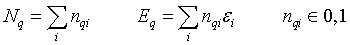Here nqi are occupation numbers that specify if microstate i is occupied in macrostate q.

To calculate the thermodynamic properties of a system of non-interacting fermions, the grand canonical partition function Zgr is constructed.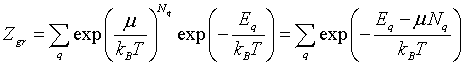The sum q runs over all of the possible macroscopic states, μ is the chemical potential, kB is Boltzmann's constant, and T is the absolute temperature. The energy and particle number of the macrostates can be expressed in terms of the microscopic states.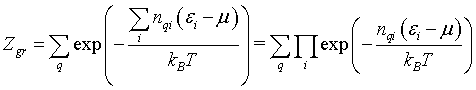The sum over all possible macrostates can also be written as the sum over all possible microstates.The microstate occupation numbers are either 0 or 1 so this can be written as,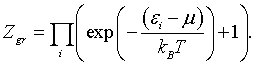The thermodynamic potential can be calculated from the grand canonical partition function, Φ = U - TS - μN = -kBTln(Zgr). Here U is the internal energy, S is the entropy, and N is the average number of fermions in the system.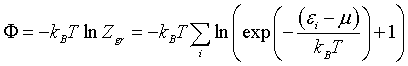This sum can be approximated by an integral over the density of states D(E). The density of states is defined per unit volume V so the grand potential density is,

$$\phi = \frac{\Phi}{V} = -k_BT\int\limits_{-\infty{}}^{\infty{}}D(E)\ln{\Bigg[\exp{\bigg({-\frac{(E-\mu)}{k_BT}}\bigg)}+1\Bigg]}\;{}dE$$

The particle density is minus the derivative of the grand potential density with respect to the chemical potential.

$$n=-\frac{\partial \phi}{\partial \mu}=\int\limits_{-\infty}^{\infty}\frac{D(E)}{1+\exp\left(\frac{E-\mu}{k_BT}\right)}dE=\int\limits_{-\infty}^{\infty}D(E)f(E)dE$$

In this expression we recognize the Fermi function, $f(E)=\left(1+\exp\left(\frac{E-\mu}{k_BT}\right)\right)^{-1}$. This is how the Fermi function is derived. Notice that it is derived for the case of non-interacting fermions. If there are interactions between the fermions (like there are between real electrons) then the Fermi function does not accurately describe the probability that a state at a given energy is occupied. Typically the electron density is known because we know the volume of the unit cell, the types of atoms in the unit cell and how many electrons each atom has. From the known electron density, the chemical potential can be calculated numerically using the above formula.

The entropy density is minus the derivative of the grand potential density with respect to the temperature.

\begin{equation} s=-\frac{\partial \phi}{\partial T}=\frac{1}{T}\int\limits_{-\infty}^{\infty}D(E)\left[\frac{E-\mu}{1+\exp\left(\frac{E-\mu}{k_BT}\right)}+k_BT\ln\left(\exp\left(-\frac{E-\mu}{k_BT}\right)+1\right)\right]dE \end{equation}

Other thermodynamic quantities can then be calculated used the standard formulas of thermodynamics. Some of these quantities are listed below. Each quantity is expressed as an integral over the density of states. On the right are buttons that link to programs that will numerically calculate the thermodynamic quantities given the electron density and a tabulated density of states. The numerical calculation proceeds by first calculating the chemical potential from the electron density and then using this value of the chemical potential to determine the other thermodynamic quantities.

 Chemical potential (implicitly defined by):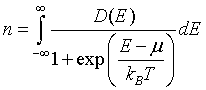Internal energy density:Energy spectral density:Grand potential density: $\phi = -k_BT\int\limits_{-\infty{}}^{\infty{}}D(E)\ln{\Bigg[\exp{\bigg({-\frac{(E-\mu)}{k_BT}}\bigg)}+1\Bigg]}\;{}dE$ Helmholz free energy density: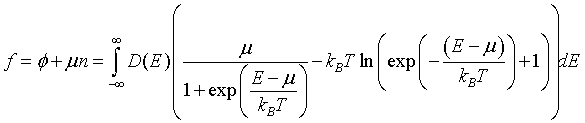Entropy density: \begin{equation} s=-\frac{\partial \phi}{\partial T}=\frac{1}{T}\int\limits_{-\infty}^{\infty}D(E)\left[\frac{E-\mu}{1+\exp\left(\frac{E-\mu}{k_BT}\right)}+k_BT\ln\left(\exp\left(-\frac{E-\mu}{k_BT}\right)+1\right)\right]dE \end{equation} Specific heat: